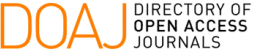### A Combinatorial Bijection between Ordered Trees and Lattice Paths

L. Rocha, E. V. Pereira Spreafico

#### Abstract

This work presents a combinatorial bijection between the set of lattice paths and the set of ordered trees, both counted by the central coefficients of the expansion of the trinomial (1+x+x^2)^n. Moreover, using a combinatorial interpretation of Catalan numbers, we establish a new set of ordered trees counted by a new sequence.

#### Keywords

lattice paths; ordered trees; combinatorial identity; central trinomial coefficients

PDF

#### References

L. P. S.Klee and R. Gillman, “On the edge set of graphs of lattice paths,” International Journal of Mathematics and Mathematical Sciences, vol. 61, p. 3291–3299, 2004.

R. Gillman, “Enumerating and constructing essentially equivalent lattice paths,” Geombinatorics, vol. 11, p. 37–42, 2001.

A. H. M. Brewer and L. Pudwell, “Graphs of essentially equivalent lattice paths,” Geombinatorics, vol. 13, p. 5–9, 2003.

N. J. A. Sloane, “The on-line encyclopedia of integer sequences,” 03 2019.

T. D. Noe, “On the divisibility of generalized central trinomial coefficients,”

Journal of Integer Sequences, vol. 9, no. 4, pp. 1–12, 2006.

T. D. Noe, “Observationes analyticae,” Novi Commentari Acad. Sci. Imper.

Petroplotanae II.Reprint in Opera Omnia, vol. 15, no. 1, pp. 50–69, 1767.

Z. Sun, “Congruences involving generalized central trinomial coefficients,” Sci.

China Math., vol. 57, p. 1375–1400, 2014.

E. Deutsch, “A bijective proof of the equation linking the schröder numbers,

large and small,” Discrete Mathematics, vol. 241, no. 1-3, pp. 235–240, 2001.

R. P. Stanley, Enumerative Combinatorics, vol. 1, second edition. Cambridge

N. Dershowitz and S. Zaks, “Enumerations of ordered trees,” Discrete Mathe- matics, vol. 31, no. 1, pp. 9–28, 1980.

DOI: https://doi.org/10.5540/tcam.2023.024.03.00427

### Refbacks

• There are currently no refbacks.

Trends in Computational and Applied Mathematics

A publication of the Brazilian Society of Applied and Computational Mathematics (SBMAC)

Indexed in: